Start typing, then use the up and down arrows to select an option from the list.# Molecular Orbital Diagrams

Pearson
120views
[AUDIO LOGO] INSTRUCTOR: As you study chemistry, you may have noticed that we use a lot of theories to try to model experimental results with some type of theory such as a Lewis structure. Lewis structures is a nice simple theory to describe the shape of a molecule. We could draw them for oxygen and nitrogen. It does a great job of predicting linear shape, and it also does very, very well in predicting that oxygen has a double bond while nitrogen has a triple bond. When we look a little bit more into some experimental data, we sometimes have to modify a theory or build on it a little bit. If we look into electronic structure, particularly magnetism, we can say that a substance is paramagnetic if it has unpaired electrons. It will also be attracted to a magnetic field. If a substance is diamagnetic, it has no unpaired electrons and it will have no attraction to a magnetic field. So based on Lewis theory and the Lewis structures of oxygen and nitrogen, what would you predict in terms of the unpaired electrons in oxygen and nitrogen? Let's take a look back at the Lewis structures for oxygen and nitrogen to see what Lewis theory would predict. When Lewis put together his theory for molecules, it's based on pairs of electrons. So in both the oxygen and the nitrogen molecule, all of our electrons are paired. Now let's come and do an experiment to see if this theory is valid. In this dewar right here I have liquid nitrogen. If we take a look at this liquid nitrogen, we can see that it's colorless. In front of me is a very powerful magnet. And we can test just to see how strong this is by taking a hammer and putting it against here. And we see that this is a lot stronger than our ordinary refrigerator magnet. So I'm going to take liquid nitrogen and now put it through the magnet to see if this liquid nitrogen is attracted to this magnetic field. As you can see, the liquid nitrogen goes straight through the magnetic field and it's not attracted at all. Now what happens when I take some liquid oxygen and do the same thing with the magnet? If we look at our liquid oxygen, we can see it has a little blue tint to it. Now we're going to take this liquid oxygen and we're going to dump it through the magnet or the magnetic field. As you can see, the oxygen is staying there in between the magnets showing that it's being attracted to the magnetic field. So based on these two experiments that we did right here, what would you predict about the magnetic properties of the oxygen and nitrogen molecule? This experiment illustrated that oxygen is paramagnetic while nitrogen is diamagnetic. Lewis theory predicted that both would be diamagnetic, so this means that we have to modify this theory or kind of take it a little bit farther. The theory that takes it to the next step is molecular orbital theory, so let's take a look at a molecular orbital diagram of oxygen and nitrogen. When we construct a molecular orbital diagram, we have the 2p and the 2s orbitals of the nitrogen and the oxygen. When we fill in these electrons in the atomic orbitals, we have to make sure that we keep the same number of electrons in the molecular orbitals. So in the 2s molecular orbital diagram, we have four electrons in an atomic orbital and we must put four electrons in the molecular orbital. Each nitrogen atom has three 2p electrons. So when we add them together to form a nitrogen molecule, we have a total of six electrons we must fill into the p-portion of the molecular orbital diagram. On the other hand, oxygen is going to be very similar in terms of the molecular orbital diagram from nitrogen. Except, remember if we look at the periodic table, oxygen has one more in the 2p orbital than nitrogen does. So that means we have to put four plus four or of eight electrons into the molecular orbital diagram. So when we do that, we fill from the bottom up to the top, and we see that oxygen is going to have two unpaired electrons here. This molecular orbital theory allows us to predict what would happen when we do the experiment and verifies this observation that oxygen is going to be paramagnetic, while nitrogen with zero unpaired electrons will be diamagnetic.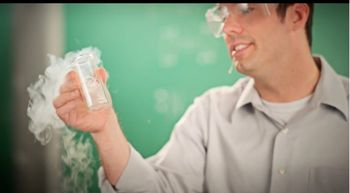05:41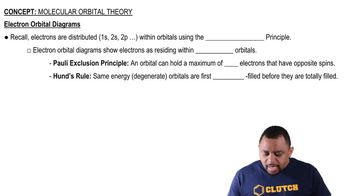01:16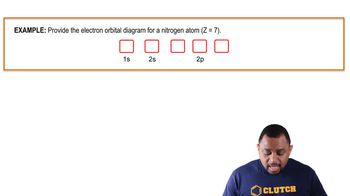00:59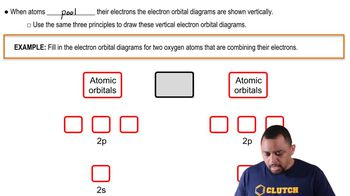01:51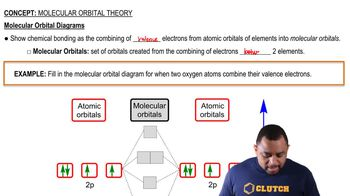03:33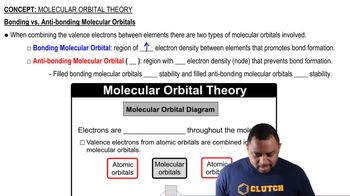02:12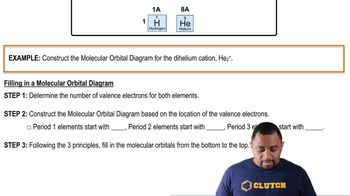01:51